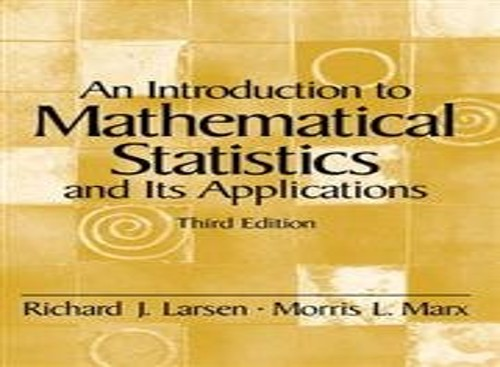# An Introduction to Mathematical Statistics and Its Applications by Richard J. Larsen

In Stock
£3.99
The third edition of this mathematical probability statistics text continues to take a calculus-based approach, and features new problems and case studies.
Only 1 left

## An Introduction to Mathematical Statistics and Its Applications Summary

### An Introduction to Mathematical Statistics and Its Applications: United States Edition by Richard J. Larsen

For courses in Mathematical Statistics.

Renowned for its high-quality, real-world case studies and examples, this highly structured text is designed to allow students with an established mathematics background to pursue a more rigorous, advanced treatment of probability and statistics. It shows HOW to use statistical methods, WHEN to use them, and reinforces the calculus that students have covered in previous courses.

### Why buy from World of BooksOur excellent value books literally don't cost the earthFree delivery in the UKEvery used book bought is one saved from landfill

(NOTE: Each chapter except Ch. 1 begins with an Introduction.)

1. Introduction.

A Brief History. Some Examples. A Chapter Summary.

2. Probability.

Sample Spaces and the Algebra of Sets. The Probability Function. Discrete Probability Functions. Continuous Probability Functions. Conditional Probability. Independence. Repeated Independent Trials. Combinatorics. Combinatorial Probability.

3. Random Variables.

The Probability Density Function. The Hypergeometric and Binomial Distributions. The Cumulative Distribution Function. Joint Densities. Independent Random Variables. Combining and Transforming Random Variables. Order Statistics. Conditional Densities. Expected Values. Properties of Expected Values. The Variance. Properties of Variances. Chebyshev's Inequality. Higher Moments. Moment-Generating Functions. Appendix 3.A.1: MINITAB Applications.

4. Special Distributions.

The Poisson Distribution. The Normal Distribution. The Geometric Distribution. The Negative Binomial Distribution. The Gamma Distribution. Appendix 4.A.1: MINITAB Applications. Appendix 4.A.2: A Proof of the Central Limit Theorem.

5. Estimation.

Estimating Parameters: The Method of Maximum Likelihood and the Method of Moments. Interval Estimation. Properties of Estimators. Minimum-Variance Estimators: The Cramer-Rao Lower Bound. Sufficiency. Consistency. Appendix 5.A.1: MINITAB Applications.

6. Hypothesis Testing.

The Decision Rule. Testing Binomial Data-H0: p = p 0. Type I and Type II Errors. A Notion of Optimality: The Generalized Likelihood Ratio.

7. The Normal Distribution.

Point Estimates for ...m and ...s2. The ...c2 Distribution; Inferences about ...s2. The F and t Distributions. Drawing Inferences about ...m. Appendix 7.A.1: MINITAB Applications. Appendix 7.A.2: Some Distribution Results for Y and S 2. Appendix 7.A.3: A Proof of Theorem 7.3.5. A Proof That the One-Sample t Test Is a GLRT.

8. Types of Data: A Brief Overview.

Classifying Data.

9. Two-Sample Problems.

Testing H 0: ...mx = ...mY-The Two-Sample t Test. Testing H0: ...s2x = ...s2Y-The F Test. Binomial Data: Testing H 0: px = py. Confidence Intervals for the Two-Sample Problem. Appendix 9.A.1: A Derivation of the Two-Sample t Test (A Proof of Theorem 9.2.2.). Appendix 9.A.2: Power Calculations for a Two-Sample t Test. Appendix 9.A.3: MINITAB Applications.

10. Goodness-of-Fit Tests.

The Multinomial Distribution. Goodness-of-Fit Tests: All Parameters Known. Goodness-of-Fit Tests: Parameters Unknown. Contingency Tables. Appendix 10.A.1: MINITAB Applications.

11. Regression.

The Method of Least Squares. The Linear Model. Covariance and Correlation. The Bivariate Normal Distribution. Appendix 11.A.1: MINITAB Applications. Appendix 11.A.2: A Proof of Theorem 11.3.3.

12. The Analysis of Variance.

The F Test. Multiple Comparisons: Tukey's Method. Testing Subhypotheses with Orthogonal Contrasts. Data Transformations. Appendix 12.A.1: MINITAB Applications. Appendix 12.A.2: A Proof of Theorem 12.2.2. Appendix 12.A.3: The Distribution of <\$E{ down 12 SSTR/ up 12 (k-1)} over { down 12 SSE/ up 12 (n-k)}> When H1 Is True.

13. Randomized Block Designs.

The F Test for a Randomized Block Design. The Paired t Test. Appendix 13.A.1: MINITAB Applications.

14. Nonparametric Statistics.

The Sign Test. The Wilcoxon Signed Rank Test. The Kruskal-Wallis Test. The Friedman Test. Appendix 14.A.1: MINITAB Applications.

Appendix: Statistical Tables.

Bibliography.

Index.

GOR005586817
An Introduction to Mathematical Statistics and Its Applications: United States Edition by Richard J. Larsen
Richard J. Larsen
Used - Good
Hardback
Pearson Education (US)
2000-06-07
790
0139223037
9780139223037
N/A
Book picture is for illustrative purposes only, actual binding, cover or edition may vary.
This is a used book - there is no escaping the fact it has been read by someone else and it will show signs of wear and previous use. Overall we expect it to be in very good condition, but if you are not entirely satisfied please get in touch with us.